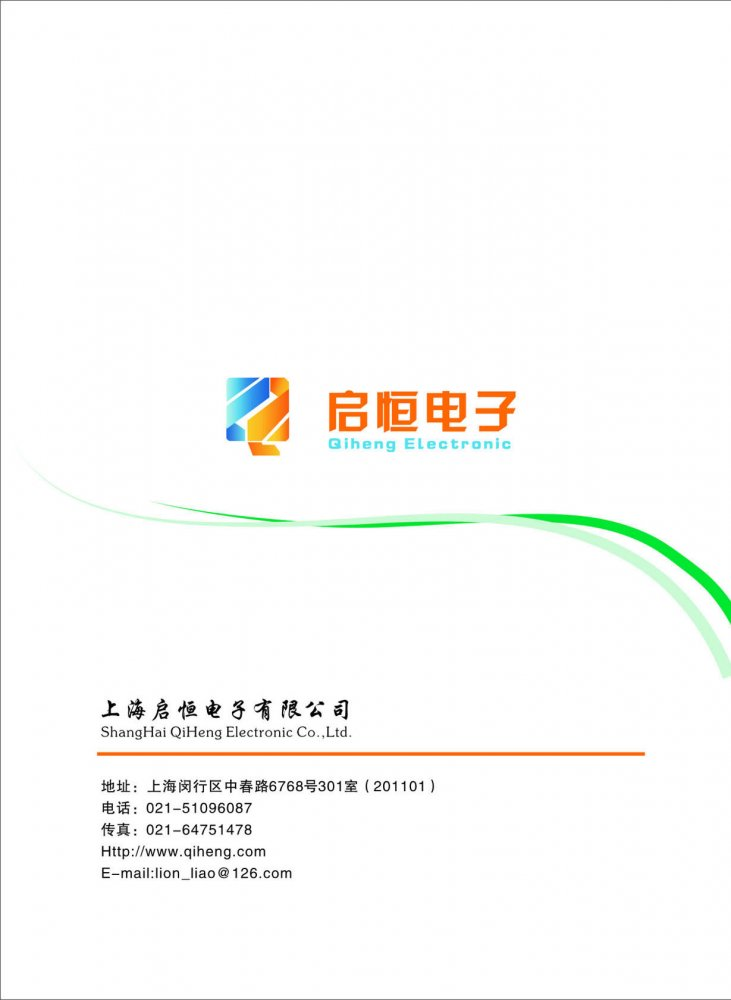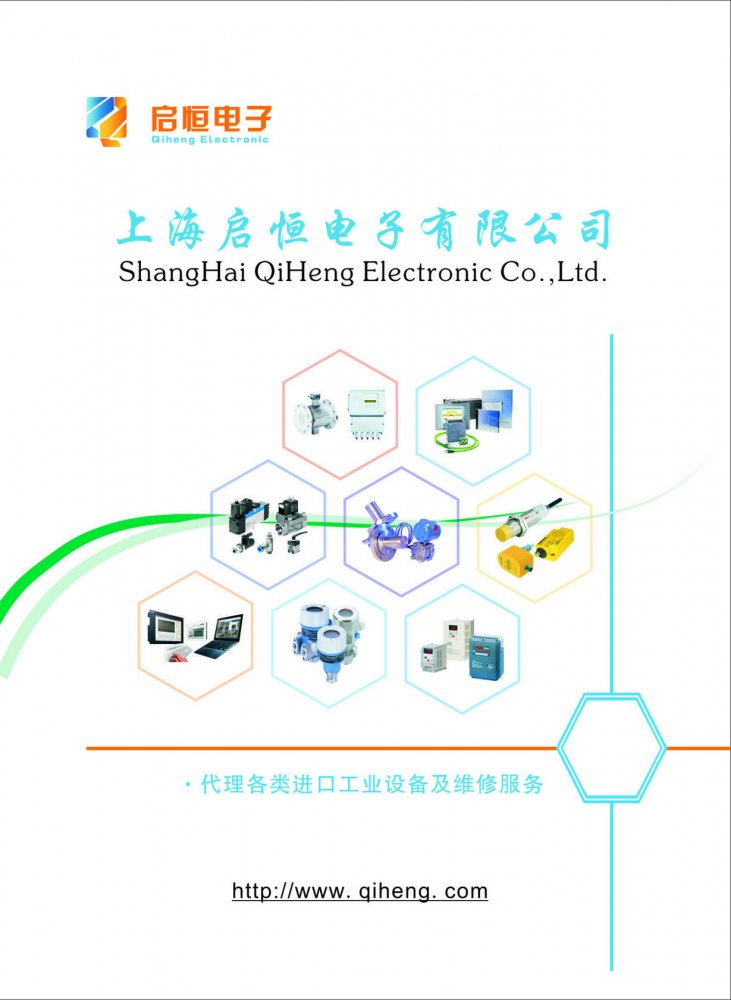• 产品信息：西门子6SE70变频器

## 西门子6SE70变频器

•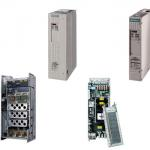•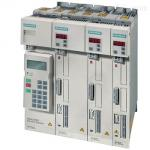•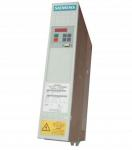产品型号： 6SE70
品  牌： 西门子
 价格电议,您可以向供应商询价得到该产品价格
所 在 地： 上海闵行区
更新日期： 2020-01-19
详细信息
西门子6se70变频器调试步骤/参数设置：一.内控参数设定 1.1 出厂参数设定 P053=7 允许CBP+PMU+PC 机修改参数 P60=2 固定设置，参数恢复到缺省 P366=0 PMU 控制 P970=0 启动参数复位 执行参数出厂设置，只是对变频器的设定与命令源进行设定，P366 参数选择不同，变频器的 设定和命令源可以来自端子，OP1S，PMU。电机和控制参数未进行设定，不能实施电机调试。 1.2 简单参数设定 P60=3 简单应用参数设置，在上述出厂参数设置的基础上，本应用设定电机控制参数 P071 进线电压(变频器400V AC / 逆变器540V DC) P95=10 IEC 电机 P100=1 V/F 开环控制 3 不带编码器的矢量控制 4 带编码器的矢量控制 P101 电机额定电压 P102 电机额定电流 P107 电机额定频率HZ P108 电机额定速度RPM P114=0 P368=0 设定和命令源为PMU+MOP P370=1 启动简单应用参数设置 P60=0 结束简单应用参数设置 执行上述参数设定后，变频器自动组合功能图连接和参数设定。P368 选择的功能图见手 册S0-S7，P100 选择的功能图见手册R0-R5。电机控制效果非*优。 1.3 系统参数设置 P60=5 P115=1 电机模型自动参数设置，根据电机参数设定自动计算 P130=10 无编码器 11 有编码器 （P151 编码器每转脉冲数） P350=电流量参考值A P351=电压量参考值V P352=频率量参考值HZ 3 3 P353=转速量参考值1/MIN P354=转矩量参考值NM P452=正向旋转*大频率或速度%（100%=P352，P353） P453=反向旋转*大频率或速度%（100%=P352，P353） P60=1 回到参数菜单，不合理的参数设置导致故障 1.4 补充参数设定如下 P128=*大输出电流A P571.1=6 PMU 正转 P572.1=7 PMU 反转 P462.1=2 从静止加速到参考频率的时间， P463=0（单位为秒S） P464.1=2 从参考频率减速到静止的时间， P465=0（S） P643.1=10V×电机*高频率/频率表*大指示 P643.2=10V×电机*大电流/电流表*大指示 P492=150% 电机转矩正限幅 P498=-150% 电机转矩负限幅 P602=1s 预励磁时间 P278=100% 无编码器速度控制中，所需*大静态转矩 P383=1000s 电机热时间常数 P384.1=150，P384.2=200 电机过载报警和停机门槛值。 1.5 调试说明 先将P100=3， P130=11 电机旋转，校验编码器的反馈波形是否正确 编码器波形正确的前提下，设定P100=4，P130=11，P151=1024。进行P115=2，4，5 的参数 优化，保证编码器矢量控制的稳定运行。 P115=2 静止状态电机辨识 P115=4 空载测试 P536=50% 速度环优化快速响应指标 P115=5 速度调节器优化 输入三个参数后均需按合闸按钮启动优化过程，该优化只适用于100=3，4 的控制方式。 与PLC通迅设置： P918 总线地址 传动反馈到PLC 的通讯字设定 P734.1=32 装置状态字1 P734.2=148 传动的速度反馈 P734.3=433 端子状态(风机/内外控) 西门子6SE70系列变频器预充电或启动时报警F002然后报警F008，测量实际电压为860V左右，但是检测着为195V，此种情况为电源板上的电压检测回路坏（有几只串联的电阻），分压板坏，更换电源板之后正常。
• 留言
标  题： *(必填)
内  容： *(必填)
联系人：  邮  箱：
手  机：  固  话：
*(必填)
公  司： *(必填)
地  址： *(必填)

• ### 联系方式

• 联系人：廖承富
• 电　话：021-51096087
• 手　机：13601664360
• 传　真：021-51096087-808
• 邮　编：201101
• 地　址：中春路6768号301
• 网　址：http://www.qiheng.com
• ### 电子样本